DIY

# Computer Voice Control Assistant< img class = "aligncenter" alt = "Assistant for computer voice control" src = "https://usamodelkina.ru/uploads/posts/2020-12/1606923607_bandicam-2020-12-02-18-37-19-561. jpg "/>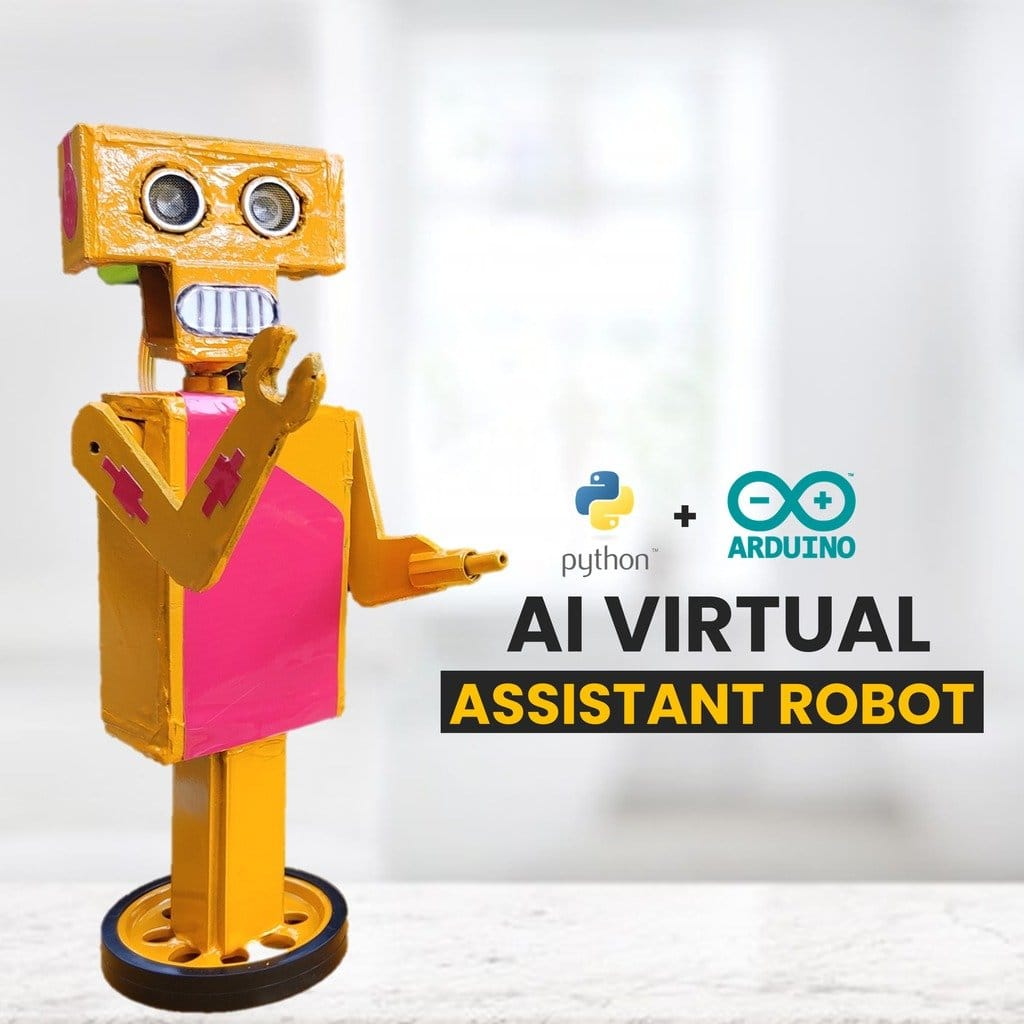In this article, the wizard will tell us how to make a virtual assistant for a computer and his physical avatar. The avatar performs some actions when you talk to him. For example, he moves his arms and head. At the end of the article you can watch a video with a demonstration of the operation and assembly of the device.
Tools and materials: -Arduino Nano; -Servo SG90 – 3pcs; -Ultrasonic sensor HC-SR04;
-PVC sheet;
-Servo wheel ( for the base);
-Knife;
-Scissors;
-Glue gun;
-Aerosol paint;
-Computer with software;
Step one: the principle of operation
The main code or part of the AI ​​(artificial intelligence) code will be executed on the computer. This is done because the computer supports the python programming language and has more processing power than the small Arduino , and also because the AI ​​bot will control/automate some of the computer's tasks. The Arduino board will be connected to the computer with a USB cable.
The idea is to run a program that will perform speech-to-text conversion, text-processing, and text-to-speech conversion. Those. a robot will listen, understand and respond. And also, its physical copy, perform some movements.
As an example, if the robot should say 'Hi/hello', the Python code will send an 'h' and then the Arduino will execute the hi () function.
Step Two: PCB
The wizard designed a PCB that can be used to create a variety of projects. It has a Micro SD card slot, a Bluetooth module slot, an external 5V power supply, and it is all powered by an Arduino Nano.
The circuit board he ordered from a specialized service, and users can download it here.Step three: diagram and installation
Then the wizard assembles the board according to the diagram.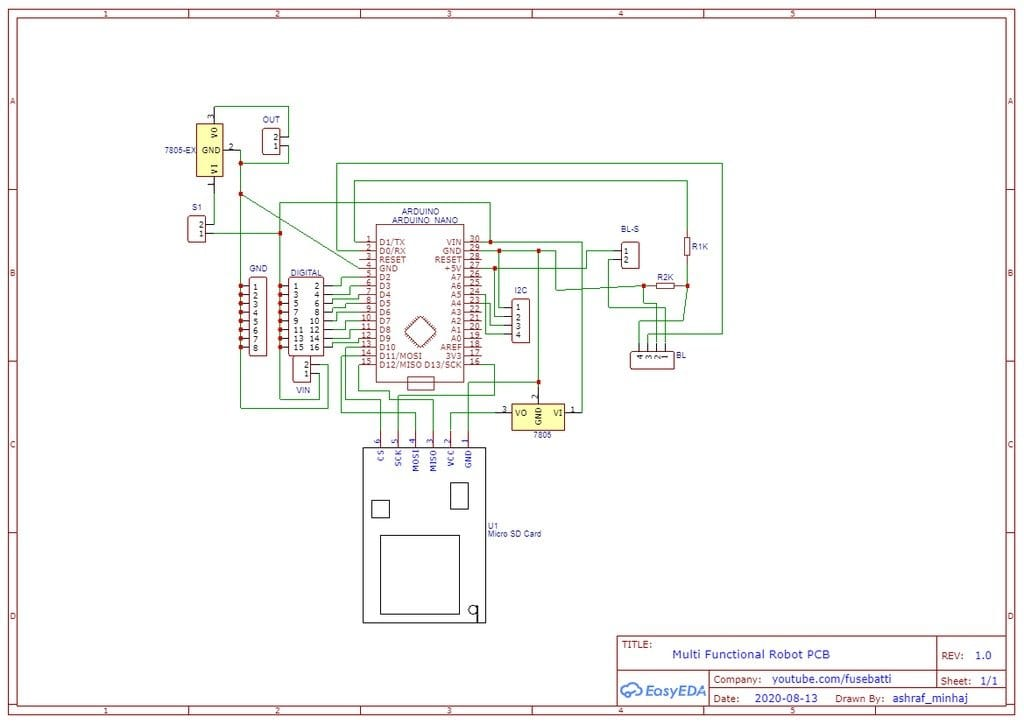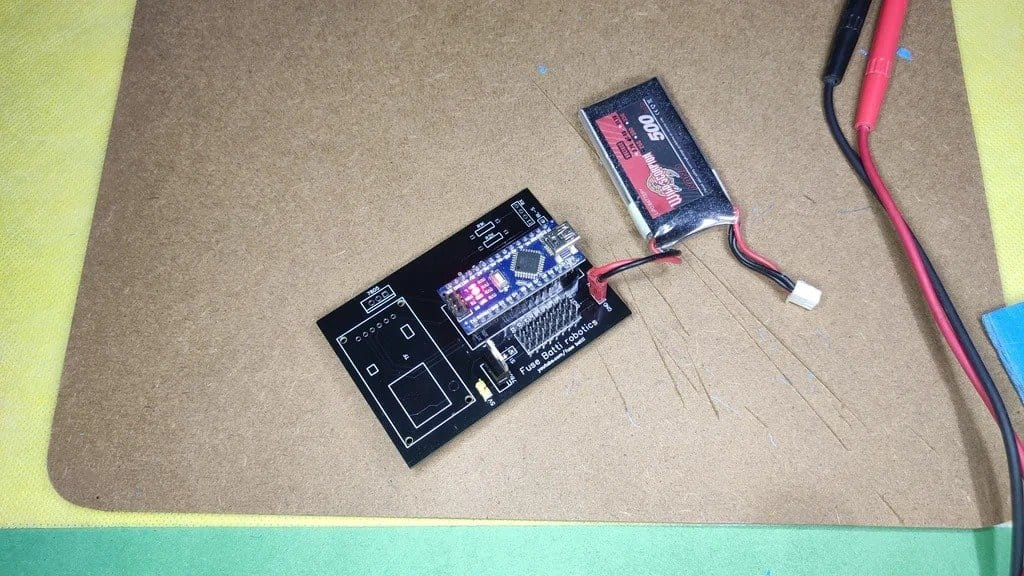Step four: assembling the bot
To make the robot's body, the master used a PVC sheet, but you can use any suitable material such as cardboard.
First, he made a torso and installed a board and servo motors in it.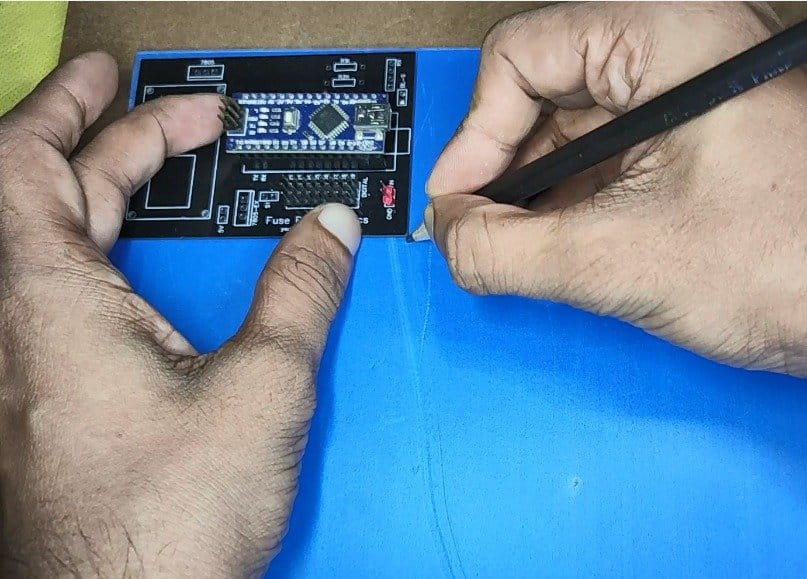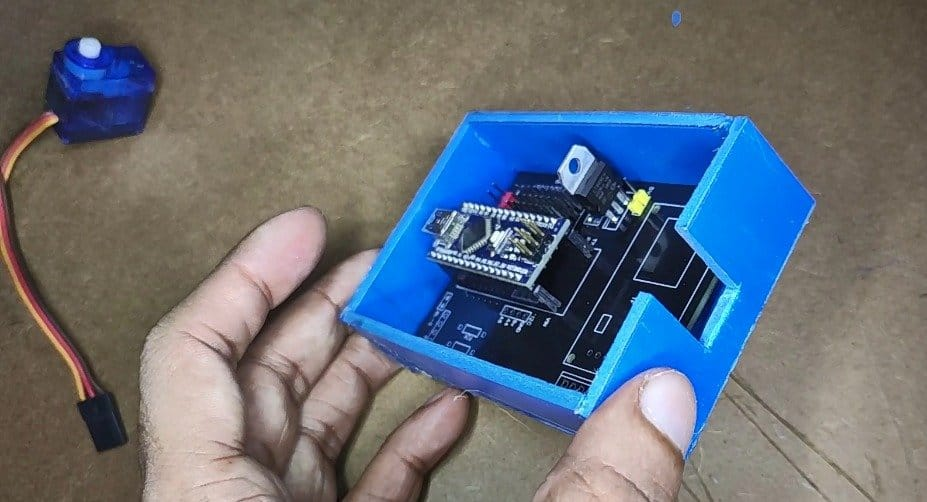One hand was made like a wrench, in the other hand I installed and glued the plug.Next made a head and installed an ultrasonic sensor in it.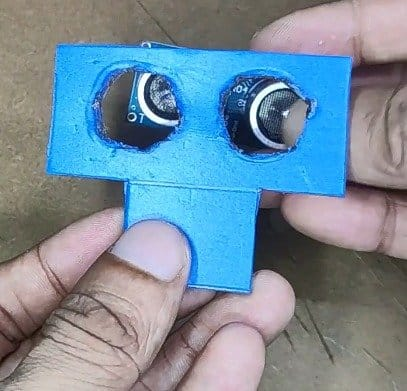I installed the servo levers, assembled the robot and painted it yellow.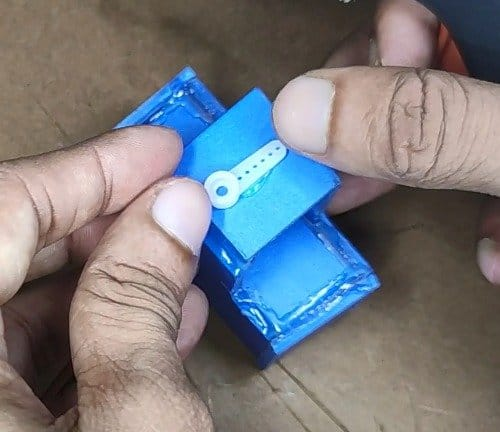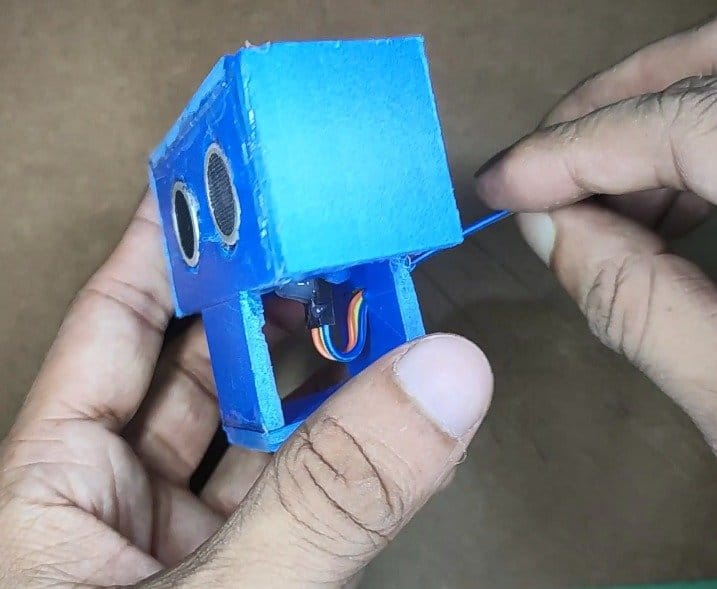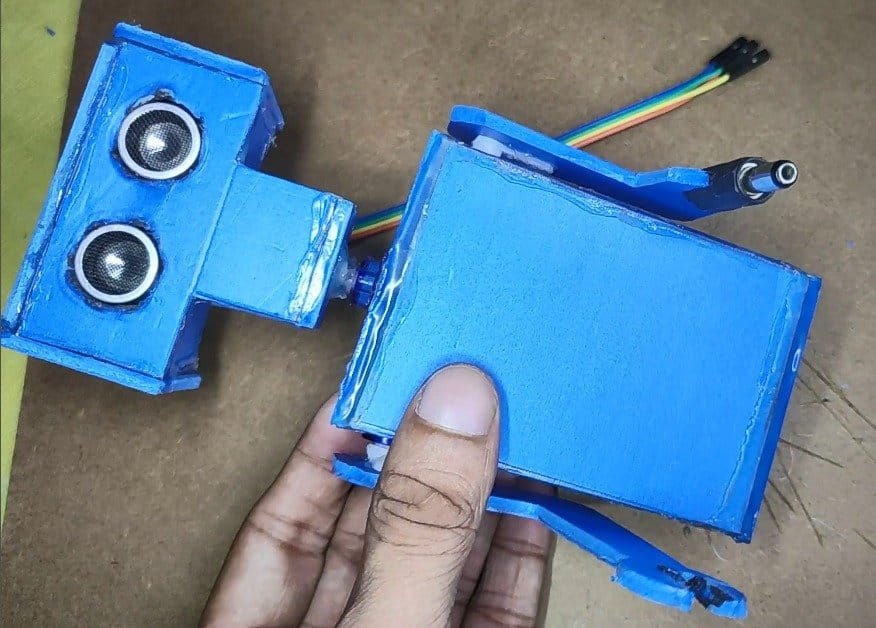Step five: programming
Any version of Python can be used, the wizard uses Python3.7.1.
After installing Python, you will need to run some commands from the command line/terminal to install the libraries for speech recognition, sound support, text-to-speech, browser automation, serial communication. We execute these commands –

` ` pip install speechrecognition pip install pyaudio pip install pyttsx3 pip install pywhatkit pip install pyserial ` `

` `author: ashraf minhaj mail: [email protected] Last Edit: Nov 2020 License: Copyright (C) Ashraf Minhaj. General Public License (GPL3 +) "" "import speech_recognition as sr # voice recognition library import random # to choose random words from list import pyttsx3 # offline Text to Speech import datetime # to get date and time import webbrowser # to open and perform web tasks import serial # for serial communication import pywhatkit # for more web automation # Declare robot name (Wake-Up word) robot_name = 'jaundice' # random words list hi_words = ['hi', 'hello', 'yo baby', 'salam '] bye_words = [' bye ',' tata ',' hasta la vista '] r_u_there = [' are you there ',' you there '] # initilize things engine = pyttsx3.init () # init text to speech engine # voices = engine.getProperty ('voices') #check for voices # engine.setProperty ('voice', voices  .id) # female voice listener = sr.Recognizer () # initialize speech recognition API # connect with NiNi motor driver board over serial communication try: port = serial.Serial ("COM15", 9600) print ("Phycial body, connected.") except: print ("Unable to connect to m y physical body ") def listen ():" "" listen to what user says "" "try: with sr.Microphone () as source: # get input from mic print (" Talk & gt; & gt; ") voice = listener. listen (source) # listen from microphone command = listener.recognize_google (voice) .lower () # use google API # all words lowercase- so that we can process easily #command = command.lower () print (command) # look for wake up word in the beginning if (command.split ('')  == robot_name): # if wake up word found .... print ("[wake-up word found]") process (command) # call process funtion to take action except: pass def process (words): "" "process what user says and take actions" "" print (words) # check if it received any command # break words in word_list = words.split (' ') [1:] # split by space and ignore the wake-up word if (len (word_list) == 1): if (word_list  == robot_name): talk ("How Can I help you?") # .write (b'l ') return if word_list  ==' play ': "" "if command for playing things, play from youtube" "" talk ("Okay boss, playing") e xtension = '' .join (word_list [1:]) # search without the command word port.write (b'u ') pywhatkit.playonyt (extension) port.write (b'l') return elif word_list  = = 'search': "" "if command for google search" "" port.write (b'u ') talk ("Okay boss, searching") port.write (b'l') extension = '' .join ( word_list [1:]) pywhatkit.search (extension) return if (word_list  == 'get') and (word_list  == 'info'): "" "if command for getting info" "" port .write (b'u ') talk ("Okay, I am right on it") port.write (b'u') extension = '' .join (word_list [2:]) # search without the command words inf = pywhatkit.info (extension) talk (inf) # read from result return elif word_list  == 'open': "" "if command for opening URLs" "" port.write (b'l ') talk ("Opening , sir ") url = f" http: //{'' .join (word_list [1:])} "# make the URL webbrowser.open (url) return elif word_list  == 'uppercut': port. write (b'U ') elif word_list  ==' smash ': port.write (b's') elif word_list  ==' punch ': port.write (b'p') # now check for matches for word in word_list: if word in hi_words: "" "if user says hi/hello greet him accordingly" "" port.write (b'h ') # send command to wave hand talk (random.choice (hi_words)) elif word in bye_words : "" "if user says bye etc" "" talk (random.choice (bye_words)) def talk (sentence): "" "talk/respond to the user" "" engine.say (sentence) engine.runAndWait () # run the app while True: listen () # runs listen one time ` `

Next, you need to program the Arduino. The wizard uses Arduino.ide to program the board.
As mentioned earlier, the Arduino program expects serial data. If it receives any data, it looks for a match. If the data matches a predefined command, it executes the command.

` `/** JAUNDICE: AI Assistant robot with Arduino and Python ** * * author: ashraf minhaj * mail: [email protected] * Last Edit: Nov 2020 * * License: Copyright (C) Ashraf Minhaj. * General Public License (GPL3 +) */# include & lt; Servo.h & gt; Servo head; Servo l_hand; Servo r_hand; //define sonar sensor's pins int trig = 4; int echo = 5; //received data byte val = ""; void setup () {//put your setup code here, to run once: head.attach (2); l_hand.attach (3); r_hand.attach (4); Serial.begin (9600); //for communicating via serial port with Python} void standby () {//all motors to these positions head.write (90); int r_pos = 30; int l_pos = map (r_pos, 0, 180, 180, 0); l_hand.write (l_pos); r_hand.write (r_pos); } void hi () {//all motors to these positions head.write (90); int i = 0; for (i = 30; i & lt; = 170; i ++) {r_hand.write (i); delay (5); } for (i = 170; i & gt; = 100; i -) {r_hand.write (i); delay (5); } for (i = 100; i & lt; = 170; i ++) {r_hand.write (i); delay (5); } for (i = 170; i & gt; = 30; i -) {r_hand.write (i); delay (5); } standby (); } void hands_up () {//do this on every command (nothing much just move hands a bit) //head.write(150); //delay (300); //head.write(90); int i = 0; for (i = 30; i & lt; = 170; i ++) {int r_pos = i; int l_pos = map (r_pos, 0, 180, 180, 0); l_hand.write (l_pos); r_hand.write (r_pos); delay (5); } delay (600); for (i = 170; i & gt; = 30; i -) {int r_pos = i; int l_pos = map (r_pos, 0, 180, 180, 0); l_hand.write (l_pos); r_hand.write (r_pos); delay (5); }} void weight_lift () {//lift weight using both hands int i = 0; for (i = 30; i & lt; = 170; i ++) {int r_pos = i; int l_pos = map (r_pos, 0, 180, 180, 0); l_hand.write (l_pos); r_hand.write (r_pos); delay (5); } for (int count = 0; count & lt; = 4; count ++) {for (i = 170; i & gt; = 60; i -) {int r_pos = i; int l_pos = map (r_pos, 0, 180, 180, 0); l_hand.write (l_pos); r_hand.write (r_pos); delay (5); } for (i = 60; i & lt; = 170; i ++) {int r_pos = i; int l_pos = map (r_pos, 0, 180, 180, 0); l_hand.write (l_pos); r_hand.write (r_pos); delay (5); }} for (i = 170; i & gt; = 30; i -) {int r_pos = i; int l_pos = map (r_pos, 0, 180, 180, 0); l_hand.write (l_pos); r_hand.write (r_pos); delay (5); }} void excited () {return; } void look_left () {//rotate hed to left head.write (180); } void confused () {for (int count = 0; count & lt; = 1; count ++) {head.write (30); r_hand.write (170); delay (700); r_hand.write (30); head.write (120); l_hand.write (30); delay (700); l_hand.write (160); } standby (); } void double_punch () {//do a punch int i = 0; for (i = 30; i & gt; = 0; i -) {int r_pos = i; int l_pos = map (r_pos, 0, 180, 180, 0); l_hand.write (l_pos); r_hand.write (r_pos); delay (5); } delay (2000); int r_pos = 80; int l_pos = map (r_pos, 0, 180, 180, 0); l_hand.write (l_pos); r_hand.write (r_pos); delay (500); standby (); } void r_upper_cut () {//make right upper-cut int i = 0; for (i = 30; i & lt; = 170; i ++) {int r_pos = i; int l_pos = map (r_pos, 0, 180, 180, 0); l_hand.write (l_pos); r_hand.write (r_pos); delay (5); } for (int count = 0; count & lt; = 4; count ++) {int i = 0; for (i = 170; i & gt; = 60; i -) {r_hand.write (i); delay (1); } for (i = 60; i & lt; = 170; i ++) {r_hand.write (i); delay (1); }} standby (); delay (100); } void smash () {//smash things int i = 0; for (i = 30; i & lt; = 170; i ++) {int r_pos = i; int l_pos = map (r_pos, 0, 180, 180, 0); l_hand.write (l_pos); r_hand.write (r_pos); delay (5); } delay (2000); for (i = 170; i & gt; = 0; i -) {int r_pos = i; int l_pos = map (r_pos, 0, 180, 180, 0); l_hand.write (l_pos); r_hand.write (r_pos); delay (1); } delay (300); int r_pos = 180; int l_pos = map (r_pos, 0, 180, 180, 0); l_hand.write (l_pos); r_hand.write (r_pos); delay (1000); standby (); } void eye_detect () {//do something if eye sensor detect motion return; } void loop () {//put your main code here, to run repeatedly: standby (); while (Serial.available () & gt; 0) //look for serial data available or not {val = Serial.read (); //read the serial value if (val == 'h') {//do hi hi (); } if (val == 'p') {//do hi double_punch (); } if (val == 'u') {hands_up (); delay (3000); } if (val == 'l') {standby (); look_left (); delay (2000); } if (val == 'U') {//uppercut r_upper_cut (); delay (2000); } if (val == 's') {smash (); delay (2000); }}} ` `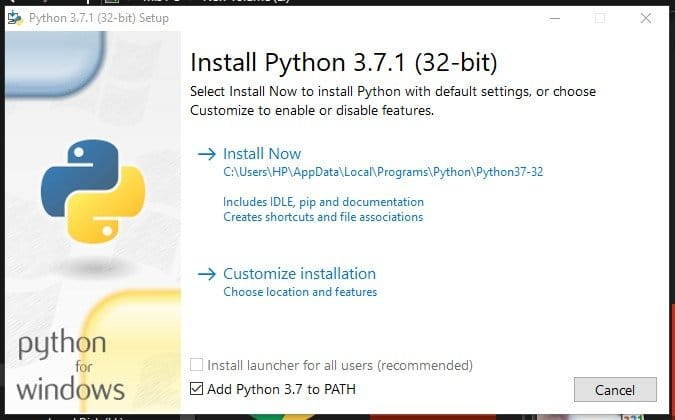After completing all these steps, the wizard connects the Arduino to the computer with a USB cable and then runs the python program. You also need to add the correct Arduino port to your Python code.Source:

usamodelkina.ru

Check Also
Close